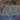## Introduction

### 为什么需要Filling Missing Values

• 为了监测环境（如空气质量、天气），大量的传感器被部署在不同的地方，进而产生了大量的Geo-sensory Time Series数据。
• 由于传感器故障和网络传输故障等原因，Geo-sensory Time Series数据往往存在大量的缺失：
• Block missing包含
• Spatial block missing：所有传感器的读数同时缺失。
• temporal block missing：某个传感器的读数在一段时间（时间长度由w给定)内出现缺失
• 2014/05/01 - 2015/04/30 Beijing### Challenge

• Readings （仪表读数） can be absent at arbitrary sensors and timestamps.

• 同时可能会出现block missing（如下图 t2 时刻所有传感器都出现了缺失），这种情况某些方法是无法处理的（比如非负矩阵分解 non-negative matrix factorization NMF)• Affected by multiple complex factors, sensor readings change over location and time significantly and non-linearly。

• 对于某个传感器的读数来说，相隔比较的远的传感器的读数可能会比相隔比较近的传感器的读数对于当前传感器更加相近，如下图，相比于S3，S1和S2更近，但是S2和S3的AQI变化却更加相似。### Contribution

• ST-MVL simultaneously considers 1) the spatial correlation between different time series and 2) the temporal correlation between readings at different timestamps in the same series, to generate a more accurate estimate.

• ST-MVL integrates the advantages of global views, i.e. empirical models derived from the data over a long period, and those of local views, i.e. data-driven algorithms that are concerned with recent readings, to achieve better accuracy.

• ST-MVL can handle the block missing problem, combining the four views in a multi-view learning

framework.

• We evaluate our method using Beijing air quality and meteorological data. The results demonstrate the advantages of our method compared with ten baselines.

## Overview

### Problem Definition

由于作者没有显式的提出Problem Definition，所以这里只是简单描述一下问题。

假设有m个传感器（记录的都是同一种数据，如PM2.5），其分别记录了长度为n的时间序列，我们可以将其表示成一个矩阵（如下图），第 i 行表示第 i 个传感器的所记录的时间序列，第 j 列表示 j 时刻所有传感器所记录的值，v_{i,j}表示第 i 个传感器第 j 时刻的读数。下图中v_{2,j}v_{1,j+1}是缺失值，我们的问题就是利用已有的信息来填充缺失值，使其尽量与真实值接近。注意：这里不同的传感器只是在不同地方而已，它们记录的是同一种数据，例如都是记录PM2.5或都是记录空气湿度。### Multi View

• 利用同一空间维度还是同一时间维度上的信息：
• spatial view：利用同一时刻空间上相邻的传感器的值去估计缺失值
• temporal view：利用同一传感器相邻时刻的值去估计缺失值
• 利用全部数据还是部分数据（这里特制是否只使用一段时间内的数据）
• global view：考虑所有时刻的数据去估计缺失值
• local view：考虑一个时间段内去估计缺失值

### Framework

u=\sum_{i=1}^n{w_i*v_i}

w_i = \frac{sim(v_i,u)}{\sum_{i=1}^n sim(v_i,u)}

u = \frac{ \sum_{i=1}^n sim(v_i,u)*vi}{\sum_{i=1}^n sim(v_i,u)}

## ST-MLV

• IDW (Inverse Distance Weighting)
• SES (Simple Exponential Smoothing)
• UCF (User-based Collaborative filtering)
• ICF (Item-based Collaborative filtering)### Global Spatial View - IDW

IDW的想法是两个传感器在空间上越靠近，那么他们所记录的时间序列应该越相似，因此我们可以使用两个传感器之间距离的倒数来作为他们的相似度，同时，为了控制权值随着距离增加的衰减速度，增加一个恒为正的指数：

IDW(v_{i,j}, v_{2,j})=distance(v_{i,j}, v_{2,j})^{-\alpha}### Global Temporal View - SES

SES的想法是一个传感器/时间序列上，越相近的两个时刻越相似。它借鉴了指数平滑模型\hat{v_{gt}}=\beta v_j + \beta (1-\beta)v_j-1 + \cdots + \beta(1-\beta)^{t_j-1}v_1，将相似度表示为：

SES(v_{2, i}, v_{2,j}) = \beta (1 - \beta)^{|j-i| - 1}### Local View### Local Spatial View - UCF

UCF是一种常见的推荐算法，它主要的想法是，相似的用户对于同一个物品的评分是相近的。对于我们这个问题，一个传感器被当成一个用户。两个传感器的相似度的计算公式为：

UCF(s_i, s) = 1 / {\sqrt{\frac {\sum_{k= j- \frac {w-1} {2}}^{ j + \frac {w+1} {2}} (v_{i,k}-v_{2,k})} {NT}}}### Local Temporal View - ICF

ICF 也是一种常见的推荐算法，它的主要想法是某个用户对相似的物品会做出相似的评分。对于我们这个问题，一个时刻被当成一个物品。则两个时刻的相似度的计算公式为：

sim(t_i, t_2) = 1 / \sqrt {\frac {\sum_{k=i}^m(v_{k,i} - v_{k, 2})} {NS}}### Multi-View Learning

\hat{v_{2,j}} = w_1 * \hat {v_{gs}} + w_2 * \hat {v_{gt}} + w_3 * \hat {v_{ls}} + w_4 * \hat {v_{lt}} + b## Experiment

### Dataset

2014/05/01 - 2015/04/30 北京的空气质量和天气数据。

### Metric

• Mean Absolute Error (MAE):

MAE = \frac {\sum_i{|v_i - \hat{v_i}|}} n
• Mean Relative Error (MRS):

MRE = \frac {\sum_i{|v_i - \hat{v_i}|}} {\sum_i v_i}

### Result## Reference

Author